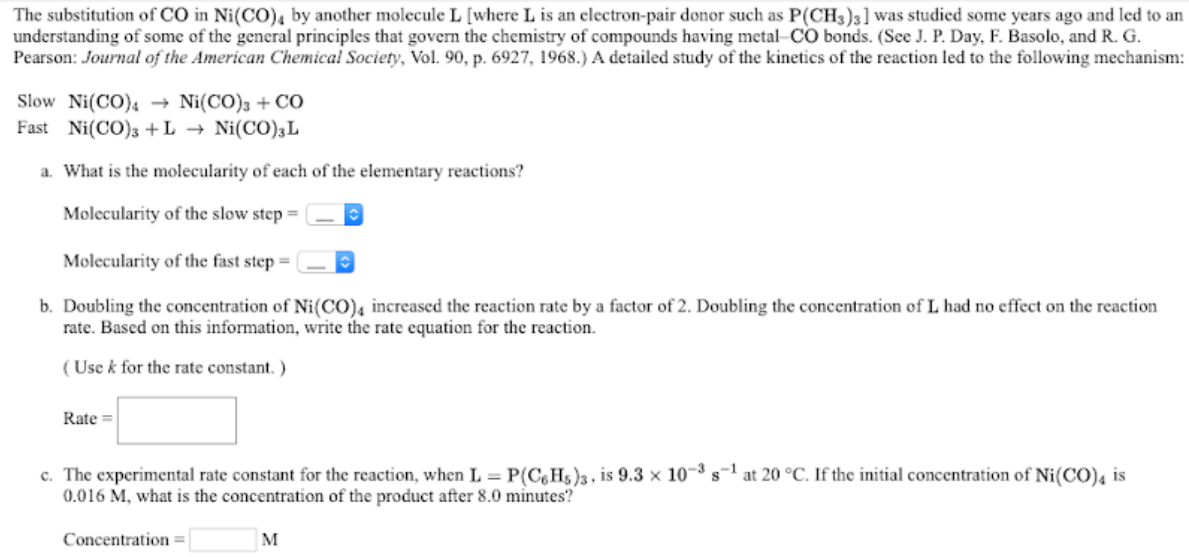# Slow Ni(CO)4 -> Ni(CO)3 + CO Fast Ni(CO)3 + L -> Ni(CO)3L a. What is the molecularity of each of the elementary reactions? b. Doubling the concentration of Ni(CO)4 increased the reaction rate by a factor of 2. Doubling the concentration of L had no effect on the reaction rate. Based on this information, write the rate equation for the reaction. c. The experimental rate constant for the reaction, when L = P(C6H5)3, is 9.3 x 10^-3 s^-1 at 20°C. If the initial concentration of Ni(CO)4 is 0.016 M, what is the concentration of the product after 8.0 minutes?